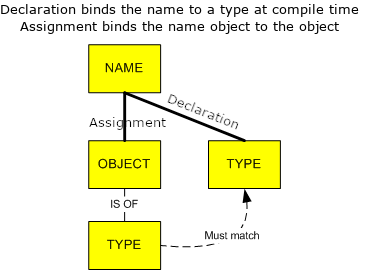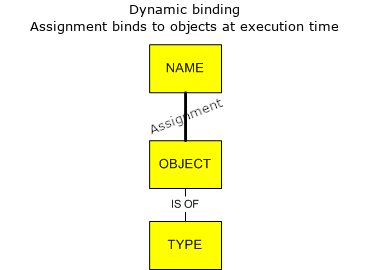# Variables¶

## Variable assignments¶

Python is a dynamical binding and typing language, contrary to C/C++, Java and Fortran, who are static binding (source: pythonconquerstheuniverse)

Static typing:Dynamic typing:Therefore, one variable name can be reused for different objects. Variable assignment is done with the = sign:

x = 1
type(x)

int

x = 2.3  # float
type(x)

float

x = 'string'  # string
type(x)

str


## Variables as objects¶

Python is object oriented. Therefore, each assigned variable is an object. Informations about the objects are accessible via the type function:

type(x)  # class of the object

str


The list of attributes and methods associated with the object are accessible via the dir function.

dir(x)  # list of methods/attributes

['__add__',
'__class__',
'__contains__',
'__delattr__',
'__dir__',
'__doc__',
'__eq__',
'__format__',
'__ge__',
'__getattribute__',
'__getitem__',
'__getnewargs__',
'__gt__',
'__hash__',
'__init__',
'__init_subclass__',
'__iter__',
'__le__',
'__len__',
'__lt__',
'__mod__',
'__mul__',
'__ne__',
'__new__',
'__reduce__',
'__reduce_ex__',
'__repr__',
'__rmod__',
'__rmul__',
'__setattr__',
'__sizeof__',
'__str__',
'__subclasshook__',
'capitalize',
'casefold',
'center',
'count',
'encode',
'endswith',
'expandtabs',
'find',
'format',
'format_map',
'index',
'isalnum',
'isalpha',
'isascii',
'isdecimal',
'isdigit',
'isidentifier',
'islower',
'isnumeric',
'isprintable',
'isspace',
'istitle',
'isupper',
'join',
'ljust',
'lower',
'lstrip',
'maketrans',
'partition',
'replace',
'rfind',
'rindex',
'rjust',
'rpartition',
'rsplit',
'rstrip',
'split',
'splitlines',
'startswith',
'strip',
'swapcase',
'title',
'translate',
'upper',
'zfill']


### Object’s attribute¶

An object’s attribute is a data which is associated with the object.

To obtain an object’s attribute, the general syntax is object.attribute. For instance, multidimensional arrays, which are numpy.array objects, have the following attributes:

import numpy as np
x = np.array([0, 1, 2, 3, 4, 5, 6])

x.dtype

dtype('int64')

x.ndim

1

x.shape

(7,)


### Object’s method¶

Methods are functions that are associated with an object, which use and eventually modify the object’s attributes.

To call an object’s method, the general syntax is object.method(arg1, arg2, ...). For instance, to compute the mean of the numpy.array defined in the above:

m = x.mean(keepdims=True)
m

array([3.])

s = x.std()
s

2.0


To get some help about a method or a function, use the help function:

help(x.mean)

Help on built-in function mean:

mean(...) method of numpy.ndarray instance
a.mean(axis=None, dtype=None, out=None, keepdims=False, *, where=True)

Returns the average of the array elements along given axis.

Refer to numpy.mean for full documentation.

--------
numpy.mean : equivalent function


### Method vs. function¶

It should be noted that object’s method are not called in the same way as module’s functions. For instance, there are two ways to compute the mean of a numpy array.

It can be done by using the mean method of the x object:

x.mean()

3.0


Or by using the mean function of the numpy module applied on the x object:

np.mean(x)

3.0

Note: In this case, the numpy function simply calls the object's method.

## Transtyping¶

To convert an object to another type:

# convert string to a list
xlist = list(x)
type(xlist)

list


## Testing object’s class¶

A usefull method is the isinstance one, which allows to determine whether an object if of a given list of class.

x = [0, 1, 2]
print(isinstance(x, (tuple)))
print(isinstance(x, (list, tuple)))

False
True


## Object’s types: mutable and immutable¶

There are of two main caterogies of objects: (source: geeksforgeeks):

• Mutable objects: Can change their contents: list, dict, set and custom objects (numpy.arrays for instance)

• Immutable objects: Can’t change their contents: int, float, bool, string, unicode, tuple}

For instance, the following statement will raise an error:

x = 'string'
# x = 1


since string are immutable, although they are close to a list object.